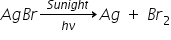# Rate of Reaction

Rate of reaction:- can be defined as the change in concentration of any one of reactant or product per unit time. R → P

Rate of reaction = Decreases in concentration of Reactant / Time taken = Increases in concentration of Product / Time taken

Rate of reaction = (Δ[R]/Δt) = (+Δ[P]/ΔT)

Units of rate of reaction are (Mol/lit)/sec = mol-1 L-1 sec-1

If gases, we take partial pressure instead of concentration then units are atm.sec-1 or mm of Hg.sec-1

Types of rate of reaction:-

•  Average rate
• Instantaneous rate
• Average rate:- The rate of reaction taken over a definite time interval.

Average rate of reaction (rav) = (Δ[R]/ Δt) = +(Δ[P]/ Δt)

• Instantaneous rate:- Rate of reaction at any particular instant of time.

Instantaneous rate of reaction (rInst) = - (d[R]/ dt) = +(d[P]/ dt)

Δ → shows  large change

d → shows  small change

Instantaneous rate of reaction is preferred over average rate because instantaneous rate of reaction gives exact values.

Expressing the rate of reaction in terms of different reactants and products

e.g. → 4 NH3 + 5 O2 → 4 NO + 6 H2O

Rate of reaction = Rate of disappearance of reactants

= - (1/4)(d[NH3]/dt) = - (1/5)(d[O2]/dt)

Rate of reaction = Rate of appearance of products

= + (1/4)(d[NO]/dt) = + (1/6)(d[NO]/dt)

For reaction → 2A + B → 3C + 4D

Rate of reaction:-   -(1/2)(dA/dt) = - (dB/dt) = + (1/3)(dC/dt) = + (1/4)(dD/dt)

• To make rate of reaction same in terms of any reactant or product, the rate of disappearance or rate of appearance is divided by stoichiometric coefficient from the balanced chemical equation.

Factors affecting the rate of reaction

1. Concentration of the reactant:- on increasing the concentration, the number of collisions increase which in turn increase rate of reaction.

2. Temperature:- On increasing the temperature, the kinetic energy of molecules increases due to which collisions increases and hence rate of reaction also increases.

3. Presence of catalyst:- Presence of catalyst increases the rate of reaction by providing an alternative pathway of  less energy requirement.

4. Nature of reactants :- If reactant changes the energy required to break the bonds the it also changes the rate of reaction

2 NO + O2 → 2 NO2 ---------- (fast)

2 CO + O2 → 2 CO2 ------------ (slow)

5. Surface area of reactant:- It affects the reaction involving solid. When solid is powdered, then it’s surface area increases, hence rate of reaction also increases. e.g.→ It is difficult to burn  a log of wood than the chips of wood.

6. Presence of light:- Light can affect only photochemical reaction because these reaction can not takes place without sunlight

For ExampleRelated Keywords